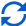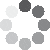Enter parameters
Enter parameters
Area Length:
ft
• inches (in)
• feet (ft)
• yards (yd)
• centimeters (cm)
• meters (m)
Area Width:
ft
• inches (in)
• feet (ft)
• yards (yd)
• centimeters (cm)
• meters (m)
Tile Length:
in
• inches (in)
• feet (ft)
• yards (yd)
• centimeters (cm)
• meters (m)
Tile Width:
in
• inches (in)
• feet (ft)
• yards (yd)
• centimeters (cm)
• meters (m)
Gap Size:
in
• inches (in)
• feet (ft)
• yards (yd)
• centimeters (cm)
• meters (m)
Waste (%):
Price:
tile
• Per Tile (tile)
• Per Box (box)
• Per square feet (ft²)
• Per square inches (in²)
• Per square yards (yd²)
• Per acres (ac)
• Per square meters ()
Box Size:Result
Tiles:
0
Area:
0
ft²
• square feet (ft²)
• square inches (in²)
• square yards (yd²)
• square miles (mi²)
• acres (ac)
• square centimeters (cm²)
• square meters ()
Сost:
$Boxes: 0(2 votes, average: 5.00 out of 5)Loading... Similar Calculators: Real-time graphics. Make the calculations and see the changes. Embed Similar Calculators:You know that, for any landscaping or home improvement project, being prepared is of paramount importance. You want to have an idea of the quantity of material required. Being accurate in your estimations and calculations is vital to the success of the project after all! That’s why we built our tile calculator. 😎 In this article we will show you how to effortlessly calculate how many tiles your next project will need. We will also explain the Mathematics behind the calculations. Contents: ## Tile calculator formulaBefore using our calculator, you must first measure the length and width of the area you wish to tile. The calculator then works out how many tiles you will require if you also provide the dimensions of the individual tiles. It does this using the formulae: $$Tiling\,Area = Area\,Length × Area\,Width$$ $$Tile\,Area = Tile\,Length × Tile\,Width$$ ### Calculating total number of tiles To calculate how many tiles you need, you will also need to determine the distance between each individual tile (i.e. the tile gap size) and the wastage of tiles (in percent): $$Number\,of\,Tiles = {Tiling\,Area \over (Tile\,Length + Gap\,Size) × (Tile\,Width + Gap\,Size)} + Waste\,\%$$ ### How to account for wastage Let’s imagine our initial calculations show that we required 50 tiles. We recommend allowing for a wastage of around 10%. Therefore, I would require another 5 tiles (10% of 50). In total, we require approximately 55 tiles. NB: Brick wastage is around 5% for professionally installed tiles, 10% if you plan to do the job yourself and 15% if the room is a complex shape. ### Calculating number of boxes required The calculator is able to work out how many boxes of tiles are required if you know how many tiles are in each box: $$Number\,of\,boxes = {Number\,of\,Tiles \over Number\,of\,Tiles\,per\,Box}$$ ### Calculating the Total Cost of the Tiling Project Finally, if you price per unit measurement then the calculator can work out the total cost of the project: $$Cost = Price\,Per\,Tile × Number\,of\,Tiles$$ or: $$Cost = Tiling\,Area × Price\,per\,Unit\,Area$$ or: $$Cost = Price\,Per\,Box × Number\,of\,Boxes$$ If you aren’t sure of the price per unit area/box/tile, you can easily calculate it: $$Price\,Per\,Tile = {Total\,Cost\,of\,Tiles \over Number\,of\,Tiles}$$ $$Price\,Per\,Unit\,Area= {Total\,Cost\,of\,Tiles \over Total\,Area\,of\,Tiles}$$ $$Price\,Per\,Box= {Total\,Cost\,of\,Boxes \over Number\,of\,Boxes}$$ Something unclear? 😕 See below for two worked examples where we work out the total number of tiles, boxes and expected cost for home improvement projects. Otherwise, simply enter your measurements into the online tile calculator to get your next project off to an efficient start! ### Example calculations • Calculate the number of shower tiles required Let’s say that I want to retile the wall in my shower. 🛁 It measures 10 feet in length and 15 feet in width. I am planning to use tiles that of size 8 inches by 5 inches, with a 0.5-inch tile gap. I will assume a brick wastage of approximately 10%. I can therefore calculate the total tiling area of the shower wall in square footage: $$Area = Area\,Length × Area\,Width = 10\,ft × 15\,ft = 150\,ft^2$$ and the individual tile area in square inches: $$Tile\,Area = Tile\,Length × Tile\,Width = 8\,in × 5\,in = 40\,in^2$$ Therefore, the total number of tiles can be worked out from the following calculations: $$Number\,of\,Tiles = {Area \over (Tile\,Length + Gap\,Size) × (Tile\,Width + Gap\,Size)} + Waste\,\% = {150\,ft^2 \over (8\,in + 0.5\,in) × (5\,in + 0.5\,in)} + 10\,\% = 508.2 \rightarrow 509\,tiles$$ Now, let’s imagine that tiles come in 25 per box and have a cost of$15 per square foot.

Therefore, I can calculate an estimate for the total cost:

$$Cost = Tiling\,Area × Price\,per\,Unit\,Area = 150\,ft^2 × 15\,/ft^2 = 2250$$

and total number of boxes required:

$$Number\,of\,boxes = {Number\,of\,Tiles \over Number\,of\,Tiles\,per\,Box} = {509 \over 25} = 20.3 \rightarrow 21\,boxes$$

Note! The actual required number of tiles and boxes is 508.2 and 20.3 respectively. It is obviously important to always round the number of tiles and boxes up. If rounded down, you will not have sufficient tiles and will need another trip to the store!

• Retiling a room calculating the price per tile

Let’s say I want to lay ceramic tiles on my bathroom floor measuring 20 feet in length and 15 feet in width.

I want to lay ceramic tiles on the bathroom floor measuring 10 inches by 7 inches, using a tile gap of 0.1 inches.

I don’t know the unit cost of the tiles, but I can purchase 50 tiles for a cost of \$375.

Therefore, I can first figure out the price per tile:

$$Price\,Per\,Tile = {Total\,Cost\,of\,Tiles \over Number\,of\,Tiles} = {375 \over 50\,tiles} = 7.5\,/tile$$

I enter these measurements into the online tile calculator, allowing for 15% wastage to calculate the tiling coverage area of the bathroom floor in square feet:

$$Area = Area\,Length × Area\,Width = 20\,ft × 15\,ft = 300\,ft^2$$

the number of tiles:

$$Number\,of\,Tiles = {Area \over (Tile\,Length + Gap\,Size) × (Tile\,Width + Gap\,Size)} + Waste\,\% = {300\,ft^2 \over (10\,in + 0.1\,in) × (7\,in + 0.1\,in)} + 15\,\% = 692.7 \rightarrow 693\,tiles$$

and an estimate for the total cost for retiling the bathroom floor with the ceramic tiles:

$$Cost = Price\,Per\,Tile × Number\,of\,Tiles = 7.5\,/tile × 693\,tiles = 5197.50$$

Finally, it’s likely that you are asking:

## What if my measurements are in various units?

The answer is simple! The estimator is able to take into account lots of different units and do the conversions for you.

Simply select the desired units in the drop down options and our calculator will do the following conversions:

$$1\,foot = 12\,inches = 0.33\,yards = 30.48\,centimeters = 0.3048\,meters$$

$$1\,ft^2 = 144\,in^2 = 0.111\,yd^2 = 929\,cm^2= 0.0929\,m^2$$

It’s that easy! 🙂

Embed this calculator on your site!Add live graphics
Copied to clipboard! Preview